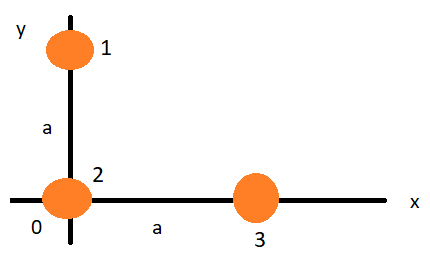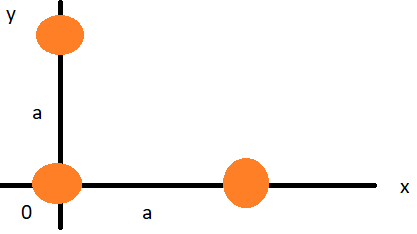# Problem: Three identical coins lie on three corners of a square a = 17.0 cm on a side, as shown in (Figure 1) .A: Determine the x coordinate of the center of gravity of the three coins. Express your answer to three significant figures and include the appropriate units.B:Determine the y coordinate of the center of gravity of the three coins. Express your answer to three significant figures and include the appropriate units.

###### FREE Expert Solution

A.

X-coordinate of the center of mass:

$\overline{){{\mathbf{x}}}_{\mathbf{c}\mathbf{m}}{\mathbf{=}}\frac{{\mathbf{m}}_{\mathbf{1}}{\mathbf{x}}_{\mathbf{1}}\mathbf{+}{\mathbf{m}}_{\mathbf{2}}{\mathbf{x}}_{\mathbf{2}}\mathbf{+}\mathbf{.}\mathbf{.}\mathbf{.}\mathbf{+}{\mathbf{m}}_{\mathbf{n}}{\mathbf{x}}_{\mathbf{n}}}{{\mathbf{m}}_{\mathbf{1}}\mathbf{+}{\mathbf{m}}_{\mathbf{2}}\mathbf{+}\mathbf{.}\mathbf{.}\mathbf{.}\mathbf{+}{\mathbf{m}}_{\mathbf{n}}}}$m1 = m2 = m3 = m

85% (4 ratings)###### Problem Details

Three identical coins lie on three corners of a square a = 17.0 cm on a side, as shown in (Figure 1) .A: Determine the x coordinate of the center of gravity of the three coins. Express your answer to three significant figures and include the appropriate units.
B:Determine the y coordinate of the center of gravity of the three coins. Express your answer to three significant figures and include the appropriate units.